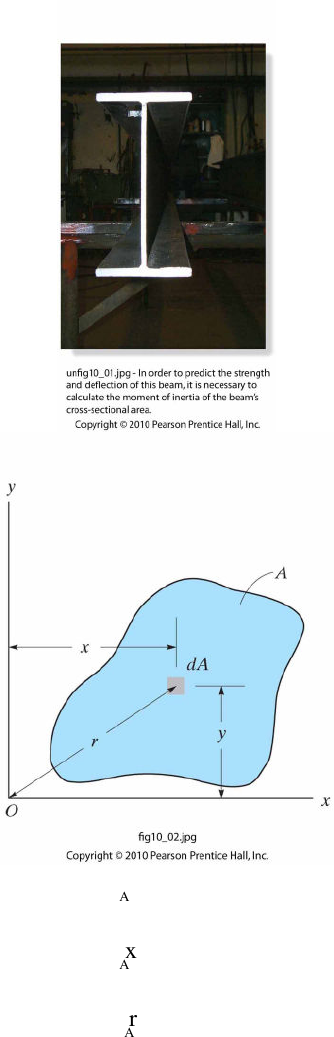Textbook Notes (270,000)
US (100,000)
LSU (20,000)
CE (800)
CE 2450 (100)
All (100)
Chapter

Student Handout Lesson 31Exam

Department
Civil Engineering
Course Code
CE 2450
Professor
All

This preview shows page 1. to view the full 4 pages of the document.Chapter(10(
Moments(of(Inertia(
(
10.1(Definition(of(Moments(of(Inertia(for(Areas(
The\$area(moment(of(inertia\$is\$a\$property\$of\$a\$cross\$section\$that\$can\$be\$used\$to\$predict\$a\$beams\$
of\$beam\$performance\$on\$the\$moment\$of\$inertia\$is\$why\$beams\$with\$high\$moments\$of\$inertia,\$such\$as\$W\$
sections\$are\$common\$in\$building\$construction\$as\$opposed\$to\$rectangular\$beams\$of\$the\$same\$area.\$
\$
\$
\$
Ix=y2dA
A
(
(
Iy=x2dA
A
(
(
Polar(Moment(of(Inertia:( ( (
JO=r2dA
A
=Ix+Iy
(
(